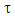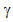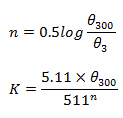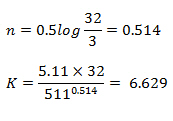# Power Law Constants (n and K) Calculation

Many types of drilling mud are classified as non-Newtonian fluid which is the behavior between Newtonian fluid model and Bingham Plastic model. The relationship between shear rate and shear stress is defined by the power law model shown below:Where= shear rate

K = consistency factor= shear rate

n = flow behavior index

n and K can be calculated from any two value of shear rate and shear stress. The method of reading shear rate on the rig comes from a V-G meter. Typically, 600 rpm, 300 rpm and 3 rpm are obtained from every mud test and we can use those reading to determine n and K.

The following equations are used to get the Power law constants (n and K).Where

n = flow behavior index, dimensionless

K = consistency factor, poise

θ300 = reading at 300 rpm

θ3 = reading at 3 rpm

Example for Power Law constants (n and K) calculation

θ300 = 32

θ3 = 3n= 0.514

K=6.629

Reference:Drilling Hydraulic Books

Share the joyWorking in the oil field and loving to share knowledge.

### 9 Responses to Power Law Constants (n and K) Calculation

1.haider says:

what we mean by Newtonian fluid

2.Martin says:

Hello,
I have a laboratory report where they ask me to calculate n and k from a log(shear stress) vs log(du/dy) graph. I know the n is the slope of the line but how would you go about finding k?

•DrillingFormulas.Com says:

K (consistency factor, poise) is derived from the equation in this article. I don’t know how to derive this equation. Most likely it comes from Eempirical relationship.

3.Antonio says:

There has been a small

tou = shear rate

K = consistency factor

gammma = shear rate

You mentioned both Tou and Gamma as shear rate.

4.Rodrick M.M says:

the value of K calculated is different to that concluded in the last line ???why

•DrillingFormulas.Com says:

It is 6.629.

5.Mario says:

I’ve being looking around for the formula to calculate n and K for the power Law. However I have found a lot of inconsistencies on the expression for K. At first I found the same as here (K=5.11*O300/511^n) on a Baker Hughes drilling fluids manual. However on different sources I have found K=510*O300/511^n (note the missing “.” on 510). These increases K by a factor of 100. I found this latter form on the Applied Drilling Engineering 1991 ed. by Bourgoyne, Millheim, etc.

•Mario says:

Never mind. Its just couse some equations are in Poise and others in centi-Poise.

Cheers.

This site uses Akismet to reduce spam. Learn how your comment data is processed.